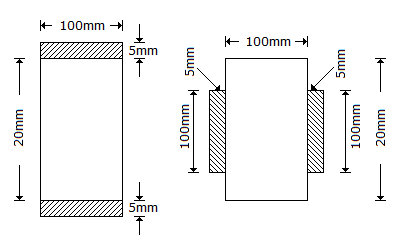# Civil Engineering - UPSC Civil Service Exam Questions

41.

What will be the curve lead for a 1 in 8 1/2 turnout taking off from a straight broad gauge track?

 A. 28.49 m B. 21.04 m C. 14.24 m D. 7.45 m

Explanation:

No answer description available for this question. Let us discuss.

42.

A timber beam of 100 mm width and 200 mm depth is reinforced with two steel plates of 100 mm width and 5 mm thickness as shown in figures.Which one of the following statements is correct for the same value of bending stress in the timber ?

 A. Moment of resistance in figure I will be more than that in figure II B. Moment of resistance in figure II will be more than that in figure I C. Moment of resistance in figure I will be equal to that in figure II D. No logical comparison can be made

Explanation:

No answer description available for this question. Let us discuss.

43.

The following statements relate to the pressure exerted by a fluid on a Submerged curved surface :
1. The vertical component of hydrostatic force acting on a submerged curved surface acts through the centre of volume of the fluid directly above the submerged area.
2. The horizontal component of the force acting on a curved surface is the hydrostatic force acting on the vertical projection of the curved surface.
3. The resultant force on a curved surface acts on the bottom of th6 curved surface.
Of these statements :

 A. 1, 2 and 3 are correct B. 2 and 3 are correct C. 1 and 2 are correct D. 1 and 3 are correct

Explanation:

No answer description available for this question. Let us discuss.

44.

The lost time due to starting delay on a traffic signal is noted to be 3s, the actual green time is 25s and yellow time is 3s. How much is the effective green time ?

 A. 31s B. 28s C. 25s D. 22s

Explanation:

No answer description available for this question. Let us discuss.

45.

Consider the following locations of a turnout:
1. Tongue rail
3. Toe of switch
4. Crossing.
Which is the correct sequence for a train to pass over the turnout from the facing direction?

 A. 3 - 1 - 2 - 4 B. 4 - 2 - 1 - 3 C. 3 - 2 - 1 - 4 D. 4 - 1 - 2 - 3